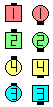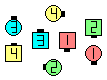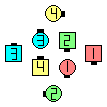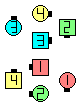Definitions of Square Dance Calls and Concepts
Wrap To A {formation} [C3A]

From Columns. EN: 10
Column \$B\$+\$i!%(B JP: 10

#1 dancer: EN: 20
1 \$BHVL\\$N?M(B: JP: 20
1/2 Split Circulate \$B\$H(B Phantom formation Circulate 2.
#2 dancer: EN: 30
2 \$BHVL\\$N?M(B: JP: 30
Circulate, 1/2 Split Circulate, \$B\$H(B Phantom formation Circulate.
#3 dancer: EN: 40
3 \$BHVL\\$N?M(B: JP: 40
Circulate 2 & 1/2.
#4 dancer: EN: 50
4 \$BHVL\\$N?M(B: JP: 50
Circulate 3.

Adjust as necessary to end in the given formationEN: 60
\$B=*\$o\$j\$GI,MW\$G\$"\$l\$P(B, \$BM?\$(\$i\$l\$?(B formation \$B\$K(B Adjust \$B\$7\$^\$9!%(B JP: 60\$B\$B;O\$a\$N(B formation:Wrap To An Hourglass\$B\$N8e(BWrap To A Galaxy\$B\$N8e(BWrap To Interlocked Diamonds\$B\$N8e(B

\$BCm
• Everyone does a total of three Circulates (or partial Circulates). EN: 70
\$BA40w\$,9g7W(B 3 \$B\$D\$N(B Circulate (\$B\$^\$?\$O(B Circulate \$B\$NItJ,(B) \$B\$r\$7\$^\$9!%(B JP: 70
• The #1 dancer always ends up as the Very Center or on the Center Line of the resulting formationEN: 80
1 \$BHVL\\$N?M\$O(B, \$B>o\$K7k2L\$N(B formation \$B\$N(B Very Center \$B\$+(B Center Line \$B>e\$G=*\$o\$j\$^\$9!%(B JP: 80
• formation can also be (Twin) Diamonds or Point-to-Point Diamonds. EN: 90
formation \$B\$K\$O(B, (Twin) Diamonds \$B\$d(B Point-to-Point Diamonds \$B\$b\$"\$j\$^\$9!%(B JP: 90
• Wrap To A formation is the Rewind of Unwrap The formation. I.e., The sequence "Wrap To A formation; U-Turn Back; Unwrap The formation; U-Turn Back" is a zero. EN: 100
Wrap To A formation \$B\$O(B Unwrap The formation \$B\$N(B Rewind \$B\$G\$9!%(B \$B\$9\$J\$o\$A(B, "Wrap To A formation; U-Turn Back; Unwrap The formation; U-Turn Back" \$B\$N(B sequence \$B\$O(B zero \$B\$G\$9!%(B \$BLuJP: 100